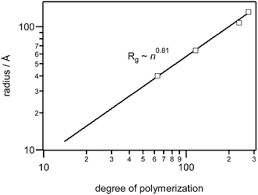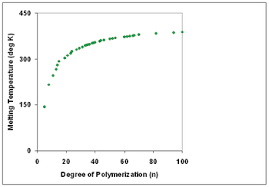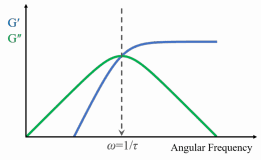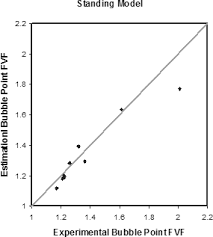## How to Calculate and Solve for Degree of Polymerization | Both Mechanisms | Polymer & TextileThe image above represents degree of polymerization | both mechanisms.

To compute for degree of polymerization, two essential parameters are needed and these parameters are parameter (σ) and parameter (V).

The formula for calculating the degree of polymerization:

Xn = 2V / 1 + σ

Where:

Xn = Degree of Polymerization
σ = Parameter
V = Parameter

Let’s solve an example;
Find the degree of polymerization when the parameter is 12 and the parameter is 18.

This implies that;

σ = Parameter = 12
V = Parameter = 18

Xn = 2V / 1 + σ
Xn = 2(18) / 1 + 12
Xn = 36 / 13
Xn = 2.769

Therefore, the degree of polymerization is 2.769.

Calculating the Parameter when the Degree of Polymerization and Parameter is Given.

V = Xn (1 + σ) / 2

Where:

V = Parameter
σ = Parameter
Xn = Degree of Polymerization

Let’s solve an example;
Find the parameter when the degree of polymerization is 22 and the parameter is 4.

This implies that;

Xn = Degree of Polymerization = 22
σ = Parameter = 4

V = Xn (1 + σ) / 2
V = 22 (1 + 4) / 2
V = 22 (5) / 2
V = 110 / 2
V = 55

Therefore, the parameter is 55.

## How to Calculate and Solve for Degree of Polymerization | combination | Polymer & TextileThe image above represents the degree of polymerization.

To compute for degree of polymerization, one essential parameter is needed and this parameter is parameter (V).

The formula for calculating the degree of polymerization:

Xn = 2V

Where:

Xn = Degree of Polymerization
V = Parameter

Let’s solve an example;
Find the degree of polymerization with a parameter of 28.

This implies that;

V = Parameter = 28

Xn = 2V
Xn = 2 (28)
Xn = 56

Therefore, the degree of polymerization is 56.

## How to Calculate and Solve for Maxwell’s Tone of Relaxation | Polymer & TextileThe image above represents maxwell’s tone of relaxation.

To compute for the maxwell’s tone of relaxation, two essential parameters are needed and these parameters are parameter (λ) and viscosity (η).

The formula for calculating the maxwell’s tone of relaxation:

G = λη

Where:

G = Maxwell’s Tone of Relaxation
λ = Parameter
η = Viscosity

Let’s solve an example;
Given that the parameter is 15 and the viscosity is 10. Find the maxwell’s tone of relaxation?

This implies that;

λ = Parameter = 15
η = Viscosity = 10

G = λη
G = (15)(10)
G = 150

Therefore, the maxwell’s tone of relaxation is 150.

Calculating the Parameter when the Maxwell’s Tone of Relaxation and the Viscosity is Given.

λ = G / η

Where;

λ = Parameter
G = Maxwell’s Tone of Relaxation
η = Viscosity

Let’s solve an example;
Given that the maxwell’s tone of relaxation is 30 and the viscosity is 15. Find the viscosity?

This implies that;

G = Maxwell’s Tone of Relaxation = 30
η = Viscosity = 15

λ = G / η
λ = 30 / 15
λ = 2

Therefore, the parameter is 2.

## How to Calculate and Solve for Standing Bubble Point Parameter | The Calculator EncyclopediaThe image above represents standing bubble point parameter.

To compute for the standing bubble point parameter, two essential parameters are needed and these parameters are API Gravity (°API) and Temperature (°Rankine) (T).

The formula for calculating standing bubble point parameter:

a = [0.00091(T – 460)] – [0.0125(°API)]

Where:

a = Standing Bubble Point Parameter, a
°API = API Gravity
T = Temperature (°Rankine)

Let’s solve an example;
Find the standing bubble point parameter when API Gravity is 32 and the temperature is 146.

This implies that:

°API = API Gravity = 32
T = Temperature (°Rankine) = 146

a = [0.00091(T – 460)] – [0.0125(°API)]
a = [0.00091(146 – 460)] – [0.0125(32)]
a = [0.00091(-314)] – [0.0125(32)]
a = [-0.28574] – [0.0125(32)]
a = [-0.28574] – [0.4]
a = -0.68574

Therefore, the standing bubble point parameter, a is -0.68574.